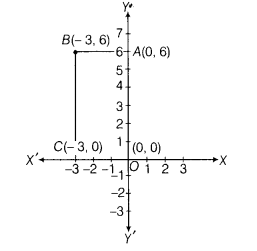# Write the coordinates of the vertices of a rectangle, whose length and breadth are 6 units and 3

Write the coordinates of the vertices of a rectangle, whose length and breadth are 6 units and 3 units respectively, one vertex at the origin, the longer side lies on the Y-axis and one of the vertices lies in the II quadrant. Also, find the area of the rectangle.

Let us draw the coordinate axes XOX’and YOY’ and choose a suitable units of distance on the axes.
Let 1cm = 1 unit.
Mark a point A on Y-axis at a 6 units distance in positive direction of Y-axis and mark a point C on X-axis at a 3 units distance in negative direction of X-axis.
Now, draw a perpendicular lines from C and A, which meets at a point B.Thus, we get the rectangle OABC whose vertices are O(0, 0), A(0, 6), B(-3, 6)andC(-3, 0)
∴ Area of rectangle = Length x Breadth
∴ Area of rectangle OABC = 6x3= 18 sq units